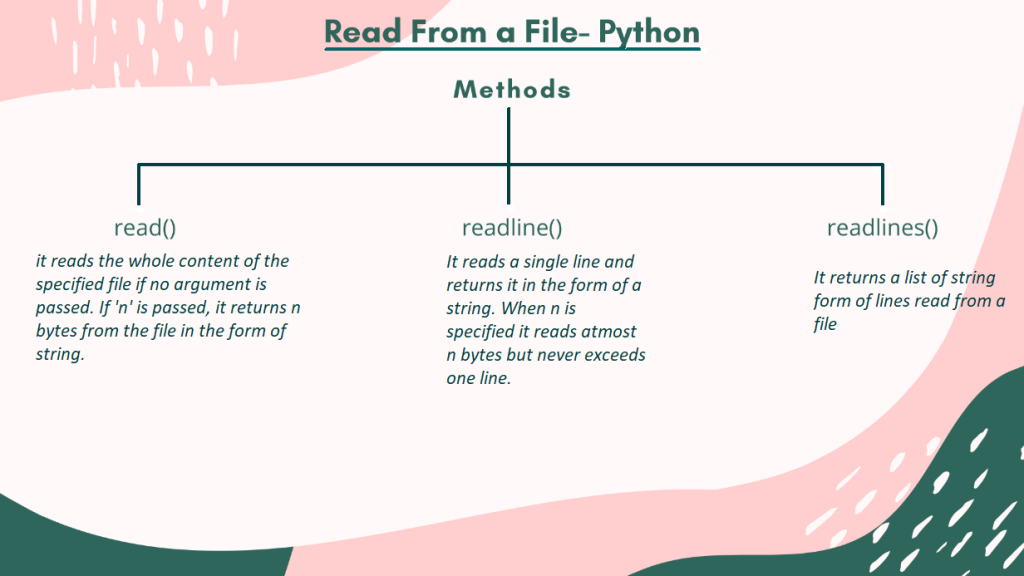# Python Read File – 3 Ways You Must KnowWe have already seen in our Python- File Handling Tutorial that how we can perform different operations in and on a file using Python programming.

One of the operations was the reading from the file, which was already created. Here we are going to elaborate on the process and look at the different methods by which we can read a file directly in Python.

## Different Methods to Read from a File in Python

Before we jump right into the methods of reading a file, we must take care of a few things. First of all, for reading, the existence of a file is very important. Secondly, the mode in which the file has been opened also matters. There are various modes in which a file can be opened in Python programming, namely,

• `r` – read-only
• `w` – only write
• `a `– append-only
• `r+` – read as well as write
• `w+` – write as well as read
• `a+` – append as well as read

Out of all the different modes available for opening a file, the file contents could be read-only in `r`, `r+`, `w+`, and `a+` modes. After we make sure that a file exists and open it in a proper readable mode, we can go further to the different functions or methods used to read file content.

The `read()` method in Python is a pre-defined function which returns the read data in the form of a string. The syntax for the `read()` method is,

Where file_open_object is the object created while opening a specific file,

and ‘n’ is the number of bytes to be read from the file. In the case where n is not specified, the `read()` function reads the whole file.

Consider the contents to be read belong to the above-shown file, named new_file.txt. Hence using `read()` we can read the information present inside new_file. Let us see how we can do that,

```file = open("new_file.txt", "r")
```

Output:

```Python
C
C++
Java
Kotlin
```

Again for reading a specific number of bytes, we can use `read()` in the following way,

```file = open("new_file.txt", "r")
```

Output:

```Python
```

`readline() `is yet another pre-defined method in Python, which returns a read line in the form of a string. Below is the syntax for `readline() `function,

Similarly, here file_open_object is the object created while opening the file and ‘n’ is the number of bytes which the function would read almost. Noteworthy, if n exceeds the length of a line, the function doesn’t consider the next line. Take a closer look at the function use,

```file = open("new_file.txt", "r")
```

Output:

```Python\n
```

Point to be noted: Here newline( `\n `) is also considered as a character.

`readlines() ` reads all the lines present inside a specified file and returns a list containing the string forms of the read lines. Given below is the syntax,

Using the `readlines()` method,

```file = open("new_file.txt", "r")
```['Python\n', 'C\n', 'C++\n', 'Java\n', 'Kotlin']